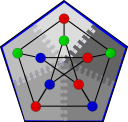#Boost C++ Libraries

...one of the most highly regarded and expertly designed C++ library projects in the world.

#Non-Distributed Betweenness Centrality

```// named parameter versions
template<typename ProcessGroup, typename Graph, typename Param, typename Tag, typename Rest>
void
non_distributed_brandes_betweenness_centrality(const ProcessGroup& pg, const Graph& g,
const bgl_named_params<Param,Tag,Rest>& params);

template<typename ProcessGroup, typename Graph, typename CentralityMap,
typename Buffer>
void
non_distributed_brandes_betweenness_centrality(const ProcessGroup& pg, const Graph& g,
CentralityMap centrality, Buffer sources);

template<typename ProcessGroup, typename Graph, typename CentralityMap,
typename EdgeCentralityMap, typename Buffer>
void
non_distributed_brandes_betweenness_centrality(const ProcessGroup& pg, const Graph& g,
CentralityMap centrality,
EdgeCentralityMap edge_centrality_map,
Buffer sources);

// non-named parameter versions
template<typename ProcessGroup, typename Graph, typename CentralityMap,
typename EdgeCentralityMap, typename IncomingMap, typename DistanceMap,
typename DependencyMap, typename PathCountMap, typename VertexIndexMap,
typename Buffer>
void
non_distributed_brandes_betweenness_centrality(const ProcessGroup& pg,
const Graph& g,
CentralityMap centrality,
EdgeCentralityMap edge_centrality_map,
IncomingMap incoming,
DistanceMap distance,
DependencyMap dependency,
PathCountMap path_count,
VertexIndexMap vertex_index,
Buffer sources);

template<typename ProcessGroup, typename Graph, typename CentralityMap,
typename EdgeCentralityMap, typename IncomingMap, typename DistanceMap,
typename DependencyMap, typename PathCountMap, typename VertexIndexMap,
typename WeightMap, typename Buffer>
void
non_distributed_brandes_betweenness_centrality(const ProcessGroup& pg,
const Graph& g,
CentralityMap centrality,
EdgeCentralityMap edge_centrality_map,
IncomingMap incoming,
DistanceMap distance,
DependencyMap dependency,
PathCountMap path_count,
VertexIndexMap vertex_index,
WeightMap weight_map,
Buffer sources);

// helper functions
template<typename Graph, typename CentralityMap>
typename property_traits<CentralityMap>::value_type
central_point_dominance(const Graph& g, CentralityMap centrality);
```

The non_distributed_betweenness_centrality() function computes the betweenness centrality of the vertices and edges in a graph. The name is somewhat confusing, the graph g is not a distributed graph, however the algorithm does run in parallel. Rather than parallelizing the individual shortest paths calculations that are required by betweenness centrality, this variant of the algorithm performs the shortest paths calculations in parallel with each process in pg calculating the shortest path from a different set of source vertices using the BGL's implementation of Dijkstra shortest paths. Each process accumulates into it's local centrality map and once all the shortest paths calculations are performed a reduction is performed to combine the centrality from all the processes.

# Where Defined

<boost/graph/distributed/betweenness_centrality.hpp>

# Parameters

IN: const ProcessGroup& pg
The process group over which the the processes involved in betweenness centrality communicate. The process group type must model the Process Group concept.
IN: const Graph& g
The graph type must be a model of the Incidence Graph concept.
IN: CentralityMap centrality

A centrality map may be supplied to the algorithm, if one is not supplied a dummy_property_map will be used and no vertex centrality information will be recorded. The key type of the CentralityMap must be the graph's vertex descriptor type.

Default: A dummy_property_map.

IN: EdgeCentralityMap edge_centrality_map

An edge centrality map may be supplied to the algorithm, if one is not supplied a dummy_property_map will be used and no edge centrality information will be recorded. The key type of the EdgeCentralityMap must be the graph's vertex descriptor type.

Default: A dummy_property_map.

IN: IncomingMap incoming

The incoming map contains the incoming edges to a vertex that are part of shortest paths to that vertex. Its key type must be the graph's vertex descriptor type and its value type must be the graph's edge descriptor type.

Default: An iterator_property_map created from a
std::vector of std::vector of the graph's edge descriptor type.
IN: DistanceMap distance

The distance map records the distance to vertices during the shortest paths portion of the algorithm. Its key type must be the graph's vertex descriptor type.

Default: An iterator_property_map created from a
std::vector of the value type of the CentralityMap.
IN: DependencyMap dependency

The dependency map records the dependency of each vertex during the centrality calculation portion of the algorithm. Its key type must be the graph's vertex descriptor type.

Default: An iterator_property_map created from a
std::vector of the value type of the CentralityMap.
IN: PathCountMap path_count

The path count map records the number of shortest paths each vertex is on during the centrality calculation portion of the algorithm. Its key type must be the graph's vertex descriptor type.

Default: An iterator_property_map created from a
std::vector of the graph's degree size type.
IN: VertexIndexMap vertex_index

A model of Readable Property Map whose key type is the vertex descriptor type of the graph g and whose value type is an integral type. The property map should map from vertices to their (local) indices in the range [0, num_vertices(g)).

Default: get(vertex_index, g)

IN: WeightMap weight_map
A model of Readable Property Map whose key type is the edge descriptor type of the graph g. If not supplied the betweenness centrality calculation will be unweighted.
IN: Buffer sources

A model of Buffer containing the starting vertices for the algorithm. If sources is empty a complete betweenness centrality calculation using all vertices in g will be performed. The value type of the Buffer must be the graph's vertex descriptor type.

Default: An empty boost::queue of int.

# Complexity

Each of the shortest paths calculations is O(V log V) and each of them may be run in parallel (one per process in pg). The reduction phase to calculate the total centrality at the end of the shortest paths phase is O(V log V).

# Algorithm Description

The algorithm uses a non-distributed (sequential) graph, as well as non-distributed property maps. Each process contains a separate copy of the sequential graph g. In order for the algorithm to be correct, these copies of g must be identical. The sources buffer contains the vertices to use as the source of single source shortest paths calculations when approximating betweenness centrality using a subset of the vertices in the graph. If sources is empty then a complete betweenness centrality calculation is performed using all vertices.

In the sequential phase of the algorithm each process computes shortest paths from a subset of the vertices in the graph using the Brandes shortest paths methods from the BGL's betweenness centrality implementation. In the parallel phase of the algorithm a reduction is performed to sum the values computed by each process for all vertices in the graph.

Either weighted or unweighted betweenness centrality is calculated depending on whether a WeightMap is passed.

Copyright (C) 2009 The Trustees of Indiana University.

Authors: Nick Edmonds and Andrew Lumsdaine